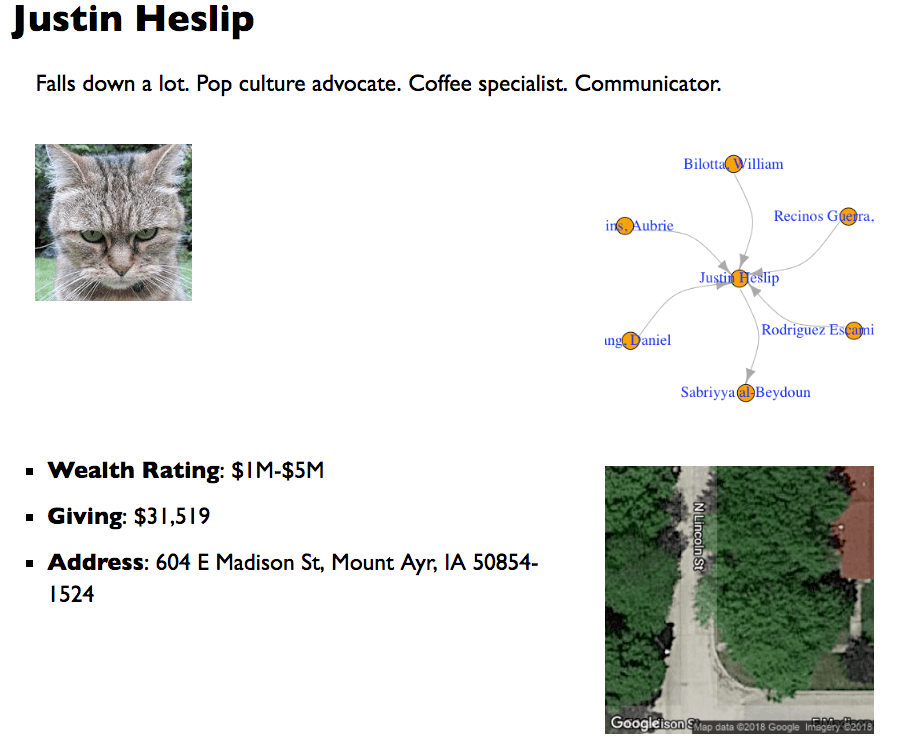Want to share your content on R-bloggers? click here if you have a blog, or here if you don't.

Have you found yourself repeating the same analysis for different groups in your data? And have you wondered about a better way of doing so in R? I have this problem often. RMarkdown, knitr, and RStudio make generating such automated reports very easy.

You need two things:

• A parent file for data prep, loading libraries and knitting the final output
• A child file with the elements you want to see on the final reportLet’s see these files in detail. You can find these files here.

# Parent or Wrapper File

This file contains scripts to load and prep data, load relevant libraries, loop to go through each group of data, and knitting the final output.

Here’s what this file looks like for this example:

```---
title: "Briefings for an important event"
output:
slidy_presentation:
keep_md: yes
css: style.css
---

```{r setup, include=FALSE}
knitr::opts_chunk\$set(echo = FALSE, warning = FALSE, message = FALSE,
cache = FALSE, fig.asp = 1)

library(tidyverse)
library(ggmap)
library(knitr)
library(igraph)

# http://www.generatorland.com/glgenerator.aspx?id=124
# https://www.fakepersongenerator.com/user-biography-generator
# https://fakena.me/fake-name/
# https://bit.ly/2INPTbT
# https://www.nature.com/articles/sdata201575
# https://dataverse.harvard.edu/dataset.xhtml?persistentId=doi:10.7910/DVN/28201

```

```{r runall, include=FALSE}
# run through each row of the dataset
# https://stackoverflow.com/a/17105758
# https://stackoverflow.com/a/19156308
out <- NULL
for (i in seq_len(nrow(bio_data))) {
out <- c(out, knitr::knit_child('indiv-briefing.Rmd'))
}
```

`r paste(out, collapse = '\n')`
```

# Child File

In this file, we specify each of the element we want to see in the report. In this example, we show photos, brief summaries, names, addresses, Google satellite view of the addresses, and a network graph. We named this file `indiv-briefing.Rmd`.

``````{r, echo=FALSE}
data <- filter(bio_data, row_number() == i)
```

# `r as.character(select(data, name))`
`r as.character(select(data, brief))`

```{r, out.width="30%"}
include_graphics("https://cataas.com/cat?type=sq")
```

```{r, echo=FALSE, message=FALSE, fig.align='left', fig.width=3.5}
par(mar = rep(0.1, 4))
network_data %>%
filter(from == i | to == i) %>%
graph_from_data_frame(d = ., vertices = filter(vertices_data, id %in% unlist(c(.\$from, .\$to), use.names = FALSE))) %>%
plot.igraph(vertex.color = "orange", vertex.label.cex = 1.2, vertex.label.color = "blue", edge.curved = TRUE)
```

- **Wealth Rating**: `r as.character(select(data, rating))`
- **Giving**: `r scales::dollar(as.numeric(select(data, giving)))`

```{r, echo=FALSE, message=FALSE, fig.align='left', fig.width=3.5}
ggmap(get_googlemap(as.character(select(data, address)), zoom = 20, maptype = "hybrid", size = c(300, 300), scale = 1), extent = "device")
```

```

Now, if you knit the parent file, you should get a report that looks something like this:Here's a video to walk through this process:

I hope this helps. What are some of the challenges you have faced while creating automated, repeatable analysis?

The post How to Create Automated Analysis Using R? appeared first on nandeshwar.info.